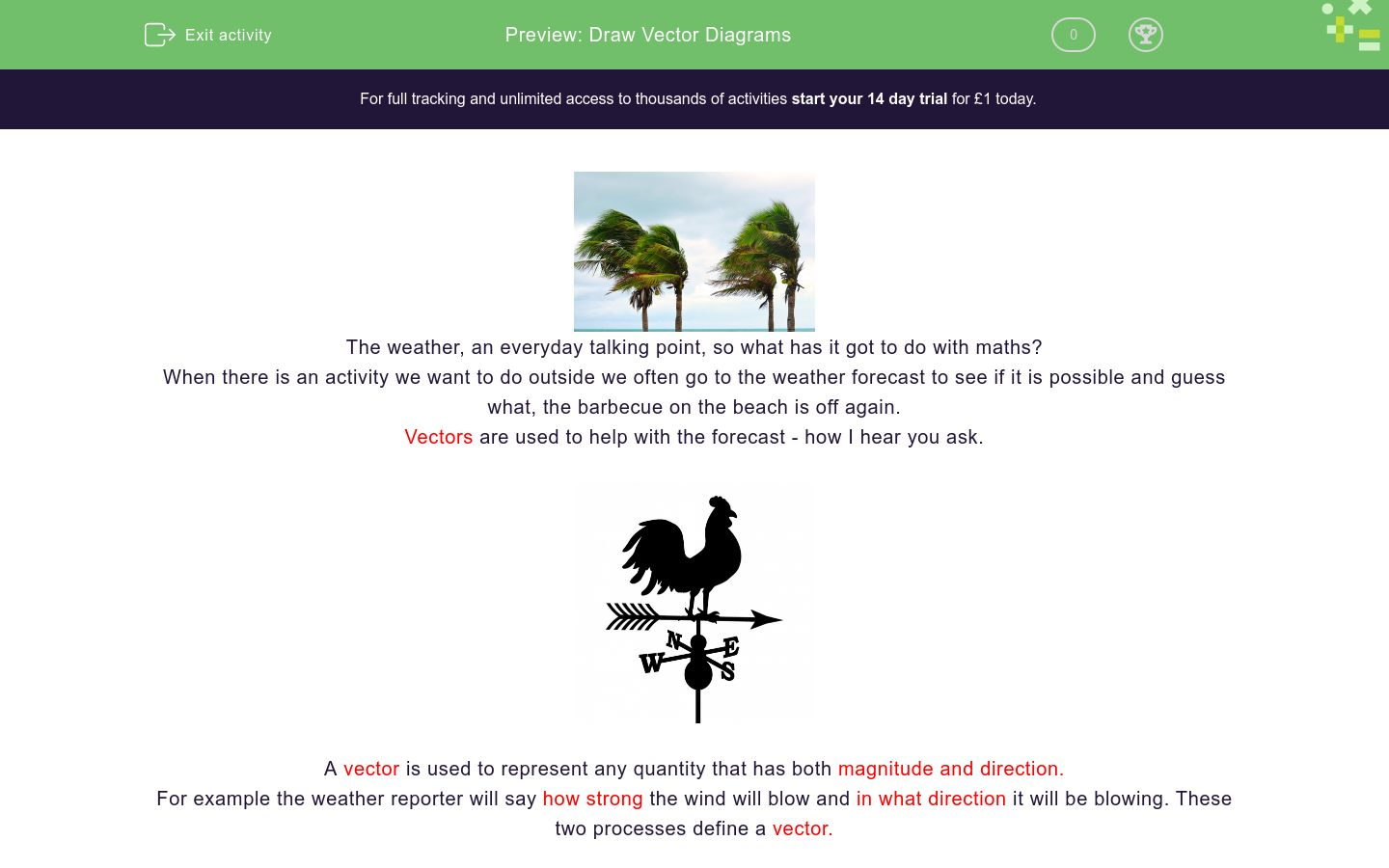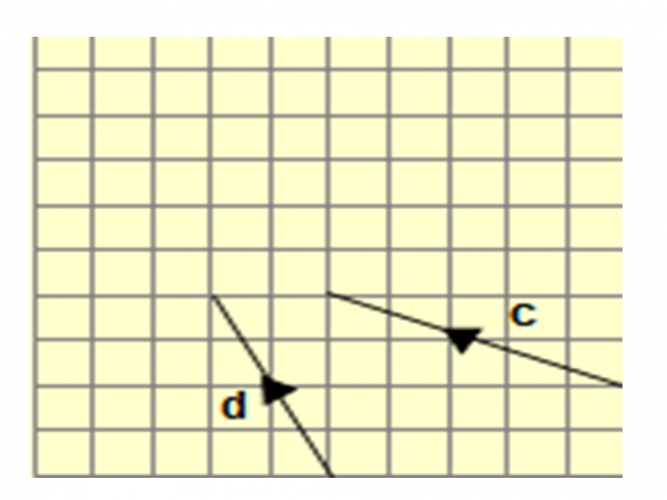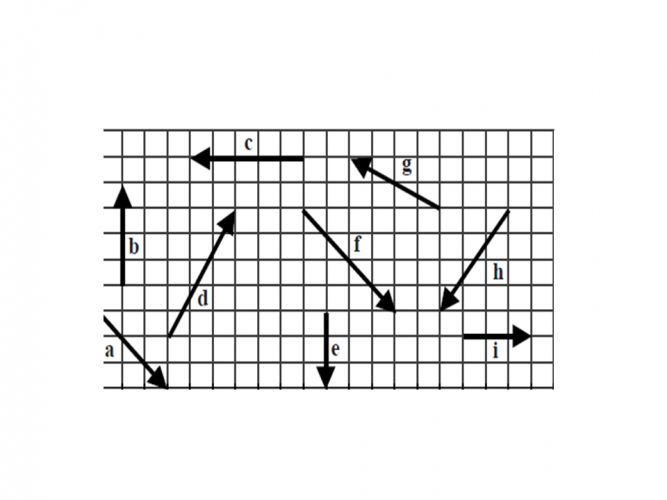# Draw Vector Diagrams

In this worksheets, students will learn how to draw vector diagrams.Key stage:  KS 4

GCSE Subjects:   Maths

GCSE Boards:   AQA, Eduqas, Pearson Edexcel, OCR

Curriculum topic:   Geometry and Measures, Congruence and Similarity

Curriculum subtopic:   Vectors, Plane Vector Geometry

Difficulty level:### QUESTION 1 of 10The weather, an everyday talking point, so what has it got to do with maths?

When there is an activity we want to do outside we often go to the weather forecast to see if it is possible and guess what, the barbecue on the beach is off again.

Vectors are used to help with the forecast - how I hear you ask.A vector is used to represent any quantity that has both magnitude and direction.

For example the weather reporter will say how strong the wind will blow and in what direction it will be blowing. These two processes define a vector.

The figures below show how vectors are represented graphically.

The arrow on the diagram shows the direction of travel.

The numbers are represented as column vectors from one point to another.

The numbers at the top represent the horizontal movement,(direction) and the numbers at the bottom represent the vertical movement(magnitude)This is the correct way to write a column vector. However, for the purpose of the activities you will need to write the as a set of

coordinates like this (-5, 2)You have drawn out the following vectors.  What column vectors were you given at the start.3, 2

2, 3

-2, -3

3 , -2What information were you given in order to draw these co-ordinates?

You will need to write your answers as a set of co-ordinates  e.g ( 2, 2)

3, 2

2, 3

-2, -3

3 , -2What column vector were you given in order to draw vector q?Vector A is  (2,4) Vector b is (-2, -3) and Vector C is (-1,-3).

 a b c Which vector has been drawn incorrectly?

Match up the diagram with the correct column vector.

Match up## Column B

a
[3,0]
b
[-4,0]
c
[2, 3]
d
[1,3]
e
[1,-3]
f
[2,-6]How would you write the column vector for c.

[4,-2]

[2, 4]

[2, -4]

[-2,-4]How would you write the column vector for f.

Column vector f is [4,4], [-4,-4], [-4,4], [4,-4].Which vector does this colum vector describe?  [3, -1]

a

b

c

d

e

f

g

hWhich vector is shown as [-4,2]Which vector is shown as [3,0]

a

b

c

d

e

f

g

h

i

• Question 1You have drawn out the following vectors.  What column vectors were you given at the start.3, 2
3 , -2
EDDIE SAYS
For you budding weather forecasters, first things first. Did you notice the direction of the arrow? This will tell you the direction of travel first. a) three units to the right and two units up (3,2) b) three units to the right and two units down (3,-2)
• Question 2What information were you given in order to draw these co-ordinates?

You will need to write your answers as a set of co-ordinates  e.g ( 2, 2)

EDDIE SAYS
It is just a matter of counting the squares. When you draw them don't be tempted to think this is easy and miscount your squares. Accuracy is the key. Always draw counting the horizontal units first and then the vertical. Movement left indicates a negative direction.
• Question 3What column vector were you given in order to draw vector q?

(2, -4)
EDDIE SAYS
These are sometimes tricky and we don't like being tricked do we. Because the arrow is pointing downwards students often think both numbers in the column vector are negative. Remember a move to the right is positive and down negative.
• Question 4Vector A is  (2,4) Vector b is (-2, -3) and Vector C is (-1,-3).

 a b c Which vector has been drawn incorrectly?
EDDIE SAYS
Did you spot this? B has been drawn as (2, - 3) If it was drawn as (2, -3) then it would have been pointing down to the left.
• Question 5

Match up the diagram with the correct column vector.

Match up## Column B

a
[-4,0]
b
[2, 3]
c
[1,3]
d
[2,-6]
e
[1,-3]
f
[3,0]
EDDIE SAYS
The direction of travel is key here. Always do the horizontal first to establish a positive or negative movement. Did you get a full house? Bingo
• Question 6How would you write the column vector for c.

[-2,-4]
EDDIE SAYS
Follow the direction of travel. Two units left [-2] and then 4 units down [ -4] Its a bit like line dancing.
• Question 7How would you write the column vector for f.

Column vector f is [4,4], [-4,-4], [-4,4], [4,-4].
EDDIE SAYS
Four units right [-4] and then 4 units down [ -4]. Top tip when the vector is pointing downwards there is going to be at least one negative number in the column vector.
• Question 8Which vector does this colum vector describe?  [3, -1]

d
EDDIE SAYS
Are you finding these okay now? On questions like this always check each one just in case there is more than one answer. You don't want to miss out on potential marks.
• Question 9Which vector is shown as [-4,2]

g
EDDIE SAYS
Hopefully you are reading these with ease now. As long as you remember along first then up or down second.
• Question 10Which vector is shown as [3,0]

i
EDDIE SAYS
These sometimes prove a little awkward because of the zero. This vector has horizontal movement therefore the first co-ordinate but no movement up of down.
---- OR ----

Sign up for a £1 trial so you can track and measure your child's progress on this activity.

### What is EdPlace?

We're your National Curriculum aligned online education content provider helping each child succeed in English, maths and science from year 1 to GCSE. With an EdPlace account you’ll be able to track and measure progress, helping each child achieve their best. We build confidence and attainment by personalising each child’s learning at a level that suits them.

Get started